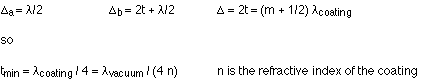## Diffraction; thin-film interference

8-3-99

### Diffraction

We discussed diffraction in PY105 when we talked about sound waves; diffraction is the bending of waves that occurs when a wave passes through a single narrow opening. The analysis of the resulting diffraction pattern from a single slit is similar to what we did for the double slit. With the double slit, each slit acted as an emitter of waves, and these waves interfered with each other. For the single slit, each part of the slit can be thought of as an emitter of waves, and all these waves interfere to produce the interference pattern we call the diffraction pattern.

After we do the analysis, we'll find that the equation that gives the angles at which fringes appear for a single slit is very similar to the one for the double slit, one obvious difference being that the slit width (W) is used in place of d, the distance between slits. A big difference between the single and double slits, however, is that the equation that gives the bright fringes for the double slit gives dark fringes for the single slit.

To see why this is, consider the diagram below, showing light going away from the slit in one particular direction.In the diagram above, let's say that the light leaving the edge of the slit (ray 1) arrives at the screen half a wavelength out of phase with the light leaving the middle of the slit (ray 5). These two rays would interfere destructively, as would rays 2 and 6, 3 and 7, and 4 and 8. In other words, the light from one half of the opening cancels out the light from the other half. The rays are half a wavelength out of phase because of the extra path length traveled by one ray; in this case that extra distance is :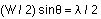The factors of 2 cancel, leaving:The argument can be extended to show that :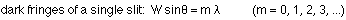The bright fringes fall between the dark ones, with the central bright fringe being twice as wide, and considerably brighter, than the rest.

### Diffraction effects with a double slit

Note that diffraction can be observed in a double-slit interference pattern. Essentially, this is because each slit emits a diffraction pattern, and the diffraction patterns interfere with each other. The shape of the diffraction pattern is determined by the width (W) of the slits, while the shape of the interference pattern is determined by d, the distance between the slits. If W is much larger than d, the pattern will be dominated by interference effects; if W and d are about the same size the two effects will contribute equally to the fringe pattern. Generally what you see is a fringe pattern that has missing interference fringes; these fall at places where dark fringes occur in the diffraction pattern.

### Diffraction gratings

We've talked about what happens when light encounters a single slit (diffraction) and what happens when light hits a double slit (interference); what happens when light encounters an entire array of identical, equally-spaced slits? Such an array is known as a diffraction grating. The name is a bit misleading, because the structure in the pattern observed is dominated by interference effects.

With a double slit, the interference pattern is made up of wide peaks where constructive interference takes place. As more slits are added, the peaks in the pattern become sharper and narrower. With a large number of slits, the peaks are very sharp. The positions of the peaks, which come from the constructive interference between light coming from each slit, are found at the same angles as the peaks for the double slit; only the sharpness is affected.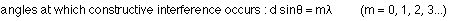Why is the pattern much sharper? In the double slit, between each peak of constructive interference is a single location where destructive interference takes place. Between the central peak (m = 0) and the next one (m = 1), there is a place where one wave travels 1/2 a wavelength further than the other, and that's where destructive interference takes place. For three slits, however, there are two places where destructive interference takes place. One is located at the point where the path lengths differ by 1/3 of a wavelength, while the other is at the place where the path lengths differ by 2/3 of a wavelength. For 4 slits, there are three places, for 5 slits there are four places, etc. Completely constructive interference, however, takes place only when the path lengths differ by an integral number of wavelengths. For a diffraction grating, then, with a large number of slits, the pattern is sharp because of all the destructive interference taking place between the bright peaks where constructive interference takes place.

Diffraction gratings, like prisms, disperse white light into individual colors. If the grating spacing (d, the distance between slits) is known and careful measurements are made of the angles at which light of a particular color occurs in the interference pattern, the wavelength of the light can be calculated.

### Thin-film interference

Interference between light waves is the reason that thin films, such as soap bubbles, show colorful patterns. This is known as thin-film interference, because it is the interference of light waves reflecting off the top surface of a film with the waves reflecting from the bottom surface. To obtain a nice colored pattern, the thickness of the film has to be similar to the wavelength of light.

An important consideration in determining whether these waves interfere constructively or destructively is the fact that whenever light reflects off a surface of higher index of refraction, the wave is inverted. Peaks become troughs, and troughs become peaks. This is referred to as a 180° phase shift in the wave, but the easiest way to think of it is as an effective shift in the wave by half a wavelength.

Summarizing this, reflected waves experience a 180° phase shift (half a wavelength) when reflecting from a higher-n medium (n2 > n1), and no phase shift when reflecting from a medium of lower index of refraction (n2 < n1).

For completely constructive interference to occur, the two reflected waves must be shifted by an integer multiple of wavelengths relative to one another. This relative shift includes any phase shifts introduced by reflections off a higher-n medium, as well as the extra distance traveled by the wave that goes down and back through the film.

Note that one has to be very careful in dealing with the wavelength, because the wavelength depends on the index of refraction. Generally, in dealing with thin-film interference the key wavelength is the wavelength in the film itself. If the film has an index of refraction n, this wavelength is related to the wavelength in vacuum by: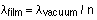### A step-by step approach

Many people have trouble with thin-film interference problems. As usual, applying a systematic, step-by-step approach is best. The overall goal is to figure out the shift of the wave reflecting from one surface of the film relative to the wave that reflects off the other surface. Depending on the situation, this shift is set equal to the condition for constructive interference, or the condition for destructive interference.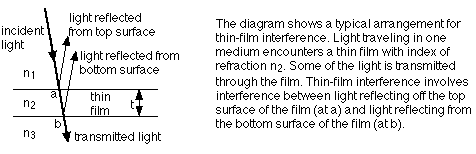Note that typical thin-film interference problems involve "normally-incident" light. The light rays are not drawn perpendicular to the interfaces on the diagram to make it easy to distinguish between the incident and reflected rays. In the discussion below it is assumed that the incident and reflected rays are perpendicular to the interfaces.

A good method for analyzing a thin-film problem involves these steps:

Step 1. Write down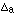, the shift for the wave reflecting off the top surface of the film.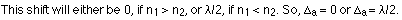Step 2. Write down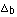, the shift for the wave reflecting off the film's bottom surface.

One contribution to this shift comes from the extra distance travelled. If the film thickness is t, this wave goes down and back through the film, so its path length is longer by 2t. The other contribution to this shift can be either 0 or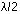, depending on what happens when it reflects (this reflection occurs at point b on the diagram).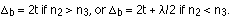Step 3. Calculate the relative shift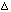by subtracting the individual shifts.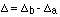Step 4. Set the relative shift equal to the condition for constructive interference, or the condition for destructive interference, depending on the situation. If a certain film looks red in reflected light, for instance, that means we have constructive interference for red light. If the film is dark, the light must be interfering destructively.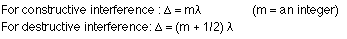Step 5. Rearrange the equation (if necessary) to get all factors of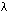on one side.

Step 6. Remember that the wavelength in your equation is the wavelength in the film itself. Since the film is medium 2 in the diagram above, we can label it. The wavelength in the film is related to the wavelength in vacuum by: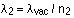Step 7. Solve. Your equation should give you a relationship between t, the film thickness, and either the wavelength in vacuum or the wavelength in the film.

### Example - a film of oil on water

Working through an example is a good way to see how the step-by-step approach is applied. In this case, white light in air shines on an oil film that floats on water. When looking straight down at the film, the reflected light is red, with a wavelength of 636 nm. What is the minimum possible thickness of the film?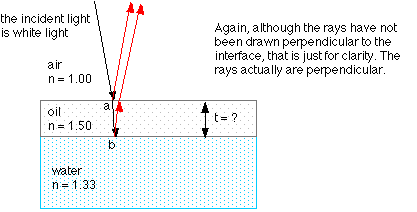Step 1. Because oil has a higher index of refraction than air, the wave reflecting off the top surface of the film is shifted by half a wavelength.Step 2. Because water has a lower index of refraction than oil, the wave reflecting off the bottom surface of the film does not have a half-wavelength shift, but it does travel the extra distance of 2t.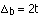Step 3. The relative shift is thus: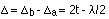Step 4. Now, is this constructive interference or destructive interference? Because the film looks red, there is constructive interference taking place for the red light.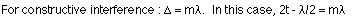Step 5. Moving all factors of the wavelength to the right side of the equation gives: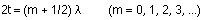Note that this looks like an equation for destructive interference! It isn't, because we used the condition for constructive interference in step 4. It looks like a destructive interference equation only because one reflected wave experienced ashift.

Step 6. The wavelength in the equation above is the wavelength in the thin film. Writing the equation so this is obvious can be done in a couple of different ways: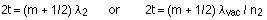Step 7. The equation can now be solved. In this situation, we are asked to find the minimum thickness of the film. This means choosing the minimum value of m, which in this case is m = 0. The question specified the wavelength of red light in vacuum, so: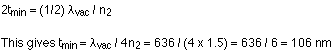This is not the only thickness that gives completely constructive interference for this wavelength. Others can be found by using m = 1, m = 2, etc. in the equation in step 6.

If 106 nm gives constructive interference for red light, what about the other colors? They are not completely cancelled out, because 106 nm is not the right thickness to give completely destructive interference for any wavelength in the visible spectrum. The other colors do not reflect as intensely as red light, so the film looks red.

### Why is it the wavelength in the film itself that matters?

The light reflecting off the top surface of the film does not pass through the film at all, so how can it be the wavelength in the film that is important in thin-film interference? A diagram can help clarify this. The diagram looks a little complicated at first glance, but it really is straightforward once you understand what it shows.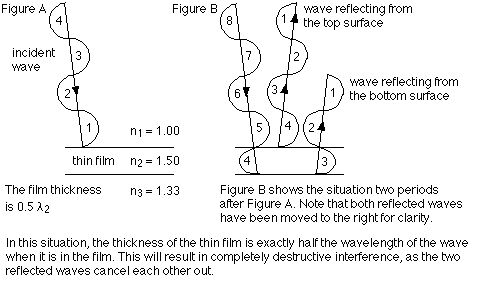Figure A shows a wave incident on a thin film. Each half wavelength has been numbered, so we can keep track of it. Note that the thickness of the film is exactly half the wavelength of the wave when it is in the film.

Figure B shows the situation two periods later, after two complete wavelengths have encountered the film. Part of the wave is reflected off the top surface of the film; note that this reflected wave is flipped by 180°, so peaks are now troughs and troughs are now peaks. This is because the wave is reflecting off a higher-n medium.

Another part of the wave reflects off the bottom surface of the film. This does not flip the wave, because the reflection is from a lower-n medium. When this wave re-emerges into the first medium, it destructively interferes with the wave that reflects off the top surface. This occurs because the film thickness is exactly half the wavelength of the wave in the film. Because a half wavelength fits in the film, the peaks of one reflected wave line up precisely with the troughs of the other (and vice versa), so the waves cancel. Destructive interference would also occur with the film thickness being equal to 1 wavelength of the wave in the film, or 1.5 wavelengths, 2 wavelengths, etc.

If the thickness was 1/4, 3/4, 5/4, etc. the wavelength in the film, constructive interference occurs. This is only true when one of the reflected waves experiences a half wavelength shift (because of the relative sizes of the refractive indices). If neither wave, or both waves, experiences a shift of, there would be constructive interference whenever the film thickness was 0.5, 1, 1.5, 2, etc. wavelengths, and destructive interference if the film was 1/4, 3/4, 5/4, etc. of the wavelength in the film.

One final philosophical note, to really make your head spin if it isn't already. In the diagram above we drew the two reflected waves and saw how they cancelled out. This means none of the wave energy is reflected back into the first medium. Where does it go? It must all be transmitted into the third medium (that's the whole point of a non-reflective coating, to transmit as much light as possible through a lens). So, even though we did the analysis by drawing the waves reflecting back, in some sense they really don't reflect back at all, because all the light ends up in medium 3.

### Non-reflective coatings

Destructive interference is exploited in making non-reflective coatings for lenses. The coating material generally has an index of refraction less than that of glass, so both reflected waves have ashift. A film thickness of 1/4 the wavelength in the film results in destructive interference (this is derived below)For non-reflective coatings in a case like this, where the index of refraction of the coating is between the other two indices of refraction, the minimum film thickness can be found by applying the step-by-step approach: